GMAT Question of the Day - Daily to your Mailbox; hard ones only

 It is currently 12 Nov 2019, 23:41### GMAT Club Daily Prep

#### Thank you for using the timer - this advanced tool can estimate your performance and suggest more practice questions. We have subscribed you to Daily Prep Questions via email.

Customized
for You

we will pick new questions that match your level based on your Timer History

Track

every week, we’ll send you an estimated GMAT score based on your performance

Practice
Pays

we will pick new questions that match your level based on your Timer History

#### Not interested in getting valuable practice questions and articles delivered to your email? No problem, unsubscribe here.Author Message
TAGS:

### Hide Tags

e-GMAT RepresentativeV
Joined: 04 Jan 2015
Posts: 3134

### Show Tags

1
1
2

Purpose of the article:

I see that you are looking to know about various types of quadrilaterals.
• Well, you have landed just at the right place.

• You will get an idea about the quadrilateral and its various types.
• You will also get to know about the properties of a few special kinds of quadrilaterals.

So, let’s get straight into it.

A quadrilateral is a polygon that has 4 sides.
• So, any closed figure that has 4 sides is a quadrilateral.
• And, all the angles of a quadrilateral sum up to 3600.

The diagram given below shows a quadrilateral ABCD and the sum of its internal angles.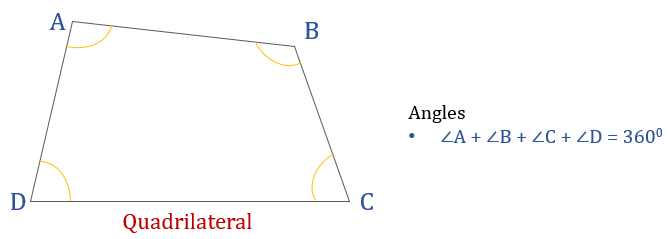There are some special kinds of quadrilateral that we see in our textbooks/ exams.
These are:
1. Rectangle
2. Square
3. Rhombus
4. Parallelogram
5. Trapezium

Let us discuss each type in detail.

Rectangle

• Each of the 4 angles are $$90^o$$
• And, opposite sides of a rectangle are equal and parallel
• Diagonals of a rectangle bisect each other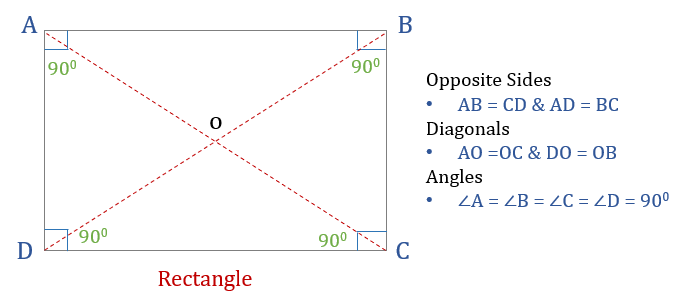Formulas to remember

If the length of the rectangle is L and breadth is B then,
1. Area of a rectangle = Length × Breadth or L × B
2. Perimeter of rectangle = 2 × (L + B)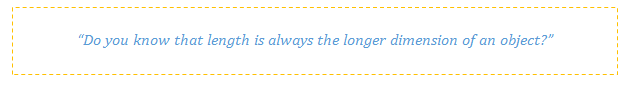Square

• That has all the angles as $$90^o$$
• All sides of a square are equal
o And, opposite sides are parallel to each other

• Diagonals bisect each other perpendicularly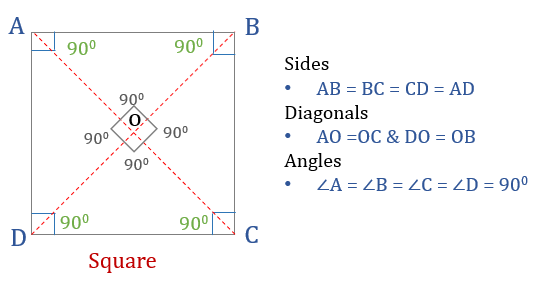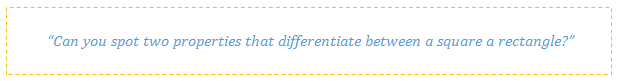Formulas to remember

If the side of a square is “a” then,
1. Area of the square = $$a × a = a^2$$
2. Perimeter of the square = 2 × (a + a) = 4a

Parallelogram

A parallelogram is a quadrilateral in which:
Opposite angles are equal
Opposite sides are equal and parallel
• Diagonals bisect each other
• Sum of any two adjacent angles is $$180^o$$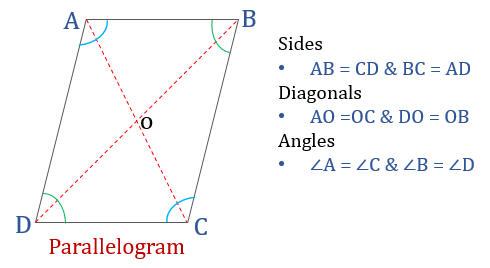Formulas to remember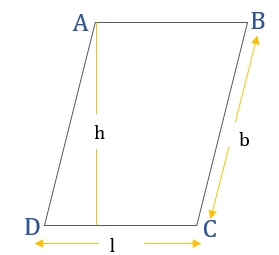If the length of a parallelogram is “l”, breadth is “b” and height is “h” then:
1. Perimeter of parallelogram= 2 × (l + b)
2. Area of the parallelogram = l × h

Rhombus

A rhombus is a quadrilateral in which:
Opposite angles are equal
All sides are equal
o And, opposite sides are parallel to each other

Diagonals bisect each other perpendicularly
• Sum of any two adjacent angles is $$180^o$$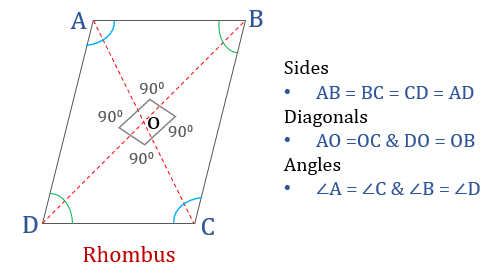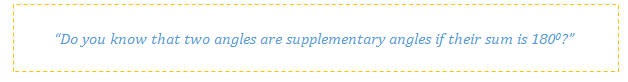Formulas to remember

If the side of a rhombus is a then,
Perimeter of rhombus= 4a

If the length of two diagonals of the rhombus is d1 and d2 then:
Area of the rhombus = $$\frac{1}{2} × d_1 × d_2$$

Trapezium

A trapezium is a quadrilateral in which:
• Only one pair of opposite sides are parallel to each other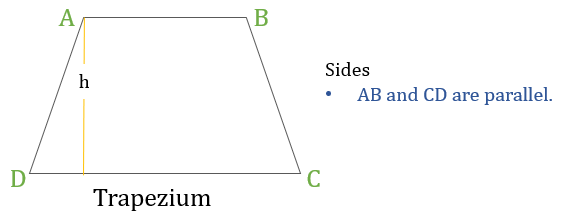Formulas to remember

If the height of a trapezium is “h” (as shown in the above diagram) then:
Perimeter of the trapezium= Sum of lengths of all the sides = AB + BC + CD + DA
Area of the trapezium = $$\frac{1}{2}$$ × (Sum of lengths of parallel sides) × h
= $$\frac{1}{2}$$× (AB + CD) × h

Summary of all the properties we learnt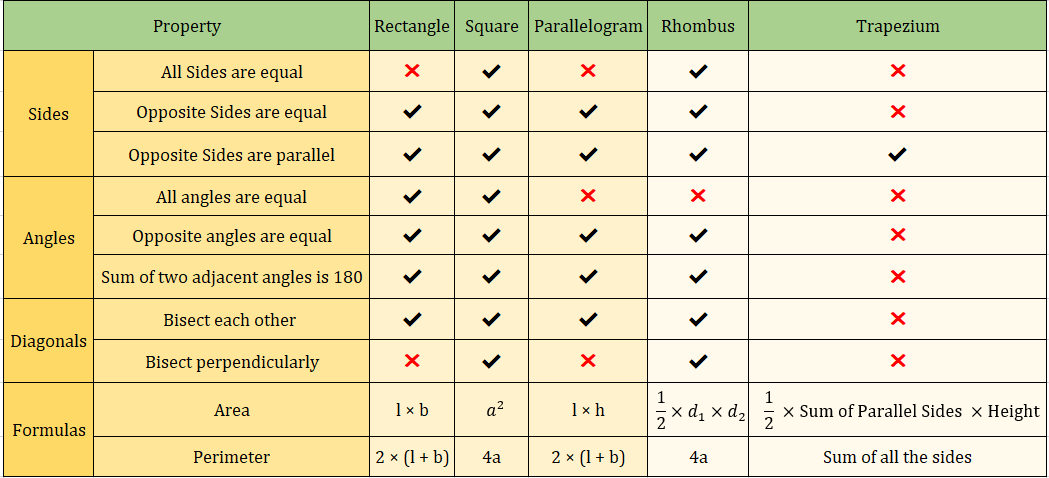_________________

Originally posted by EgmatQuantExpert on 10 Jul 2019, 01:00.
Last edited by EgmatQuantExpert on 23 Jul 2019, 23:47, edited 2 times in total.
InternB
Joined: 21 Oct 2018
Posts: 32
Location: India

### Show Tags

Practice test is missing EgmatQuantExpert and great article though
e-GMAT RepresentativeV
Joined: 04 Jan 2015
Posts: 3134

### Show Tags

1
dine5207 wrote:
Practice test is missing EgmatQuantExpert and great article though

Hey dine5207,
Glad that you liked the article.

We will update the quizzes link soon in the article.

Regards,
Ashutosh
_________________
e-GMAT RepresentativeV
Joined: 04 Jan 2015
Posts: 3134

### Show Tags

Hey Everyone,

Exercise Questions

Detailed solutions will be posted soon.

Happy Learning!_________________
e-GMAT RepresentativeV
Joined: 04 Jan 2015
Posts: 3134

### Show Tags

Hey everyone,

The answers to the practice questions have been posted.

Regards,
e-GMAT
_________________Display posts from previous: Sort by